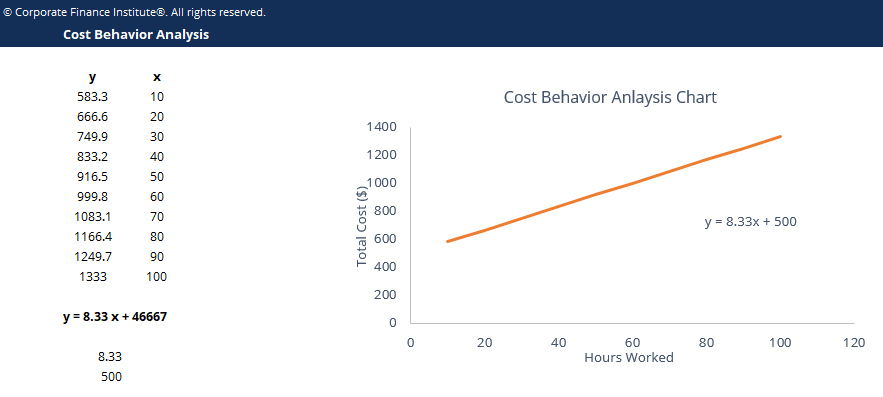# Cost Behavior Analysis Template

## Cost Behavior Analysis Template

This cost behavior analysis template shows you how a cost changes with changes in the level of activity relating to that cost.

Below is a screenshot of the cost behavior analysis template:### Download the Free Template

Enter your name and email in the form below and download the free template now!

### Cost Behavior Analysis Template

Download the free Excel template now to advance your finance knowledge!

Cost behavior analysis refers to management’s attempt to understand how operating costs change in relation to a change in an organization’s level of activity. These costs may include direct materials, direct labor, and overhead costs that are incurred from developing a product. Management typically performs cost behavior analysis through mathematical cost functions.

Cost functions are descriptions of how a cost (i.e. material, labor, or overhead) changes with changes in the level of activity relating to that cost. For example, total variable costs will change in relation to increased activity, while fixed costs will remain the same. Cost functions may come in various forms.

### Cost function assumptions

Cost functions are usually given in the form of y = mx + b and can be plotted on a graph.

In order to determine these cost functions, managers typically make the following assumptions for simplicity purposes:

• Variations in the cost driver explain the variations in the related total costs.
• Cost behavior can be summarized into a linear cost function within a relevant range.

### More Free Templates

For more resources, check out our business templates library to download numerous free Excel modeling, PowerPoint presentation and Word document templates.

• Excel Modeling Templates
• PowerPoint Presentation Templates
• Transaction Document Templates

### Financial Analyst Certification

Become a certified Financial Modeling and Valuation Analyst (FMVA)® by completing CFI’s online financial modeling classes and training program!